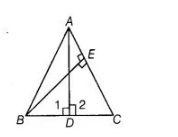# If ABC is an isosceles triangle in`
Question:

If ABC is an isosceles triangle in which AC = BC, AD and BE are respectively two altitudes to sides BC and AC, then prove that AE = BD.

Solution:

Given $\triangle A B C$ is an isosceles triangle in which $A C=B C$. Also, $A D$ and $B E$ are two altitudes to sides $B C$ and $A C$, respectively.

To prove $A E=B D$.

Proof In $\triangle A B C$,    $A C=B C$     [given]

$\angle A B C=\angle C A B$

[angles opposite to equal sides are equal]i.e., $\angle A B D=\angle E A B$ $\ldots($ i)

In $\triangle A E B$ and $\triangle B D A$,

$\angle A E B=\angle A D B=90^{\circ}$ [given, $A D \perp B C$ and $B E \perp A C$ ]

$\angle E A B=\angle A B D$ [from Eq. (1)]

and $A B=A B$ [common side]

$\therefore$ $\triangle A E B \cong \triangle B D A$ [by AAS congruence rule]

$\Rightarrow$ $A E=B D$ [by CPCT]

Hence proved.